# estimate

Fit threshold-switching dynamic regression model to data

Since R2021b

## Syntax

``EstMdl = estimate(Mdl,tt0,Y)``
``EstMdl = estimate(Mdl,tt0,Y,Name,Value)``
``````[EstMdl,logL] = estimate(___)``````
``````[EstMdl,logL] = estimate(ax,___)``````
``````[EstMdl,logL,h] = estimate(ax,___)``````

## Description

example

````EstMdl = estimate(Mdl,tt0,Y)` estimates parameters of the threshold-switching dynamic regression model `Mdl`. `estimate` fits the model to the response data `Y`, and initializes the estimation procedure by treating the parameter values of the fully specified threshold transitions `tt0` as initial values. `estimate` implements a version of the conditional least-squares algorithm described in .```

example

````EstMdl = estimate(Mdl,tt0,Y,Name,Value)` uses additional options specified by one or more name-value arguments. For example, `estimate(Mdl,tt0,Y,IterationPlot=true)` displays a plot of the loglikelihood versus the iteration step, after the algorithm terminates.```

example

``````[EstMdl,logL] = estimate(___)``` also returns the estimated loglikelihood `logL` when the algorithm terminates, using any of the input argument combinations in the previous syntaxes.```
``````[EstMdl,logL] = estimate(ax,___)``` plots iterations on the axes specified by `ax` instead of the current axes (`gca`). The option `ax` can precede any of the input argument combinations in the previous syntaxes.```
``````[EstMdl,logL,h] = estimate(ax,___)``` also returns the plot handle `h`. Use `h` to modify properties of the plot after you create it.```

## Examples

collapse all

Assess estimation accuracy using simulated data from a known data-generating process (DGP). This example uses arbitrary parameter values.

Create Model for DGP

Create a discrete threshold transition at mid-level `1`.

`ttDGP = threshold(1)`
```ttDGP = threshold with properties: Type: 'discrete' Levels: 1 Rates: [] StateNames: ["1" "2"] NumStates: 2 ```

`ttDGP` is a `threshold` object representing the state-switching mechanism of the DGP.

Create the following fully specified self-exciting TAR (SETAR) model for the DGP.

• State 1: ${\mathit{y}}_{\mathit{t}}={\epsilon }_{\mathit{t}}$.

• State 2: ${\mathit{y}}_{\mathit{t}}=2+{\epsilon }_{\mathit{t}}$.

• ${\epsilon }_{\mathit{t}}\sim Ν\left(0,1\right)$.

Specify the submodels by using `arima`.

```mdl1DGP = arima(Constant=0); mdl2DGP = arima(Constant=2); mdlDGP = [mdl1DGP mdl2DGP];```

Because the innovations distribution is invariant across states, the `tsVAR` software ignores the value of the submodel innovations variance (`Variance` property).

Create a threshold-switching model for the DGP. Specify the model-wide innovations variance.

`MdlDGP = tsVAR(ttDGP,mdlDGP,Covariance=1);`

`MdlDGP` is a `tsVAR` object representing the DGP.

Simulate Response Paths from DGP

Generate a random response path of length 100 from the DGP. By default, `simulate` assumes a SETAR model with delay $\mathit{d}=1$. In other words, the threshold variable is ${\mathit{y}}_{\mathit{t}-1}$.

```rng(1) % For reproducibiliy y = simulate(MdlDGP,100);```

`y` is a 100-by-1 vector of representing the simulated response path.

Create Model for Estimation

Create a partially specified threshold-switching model that has the same structure as the data-generating process, but specify the transition mid-level, submodel coefficients, and model-wide constant as unknown for estimation.

```tt = threshold(NaN); mdl1 = arima('Constant',NaN); mdl2 = arima('Constant',NaN); Mdl = tsVAR(tt,[mdl1,mdl2],'Covariance',NaN);```

`Mdl` is a partially specified `tsVAR` object representing a template for estimation. `NaN`-valued elements of the `Switch` and `Submodels` properties indicate estimable parameters.

`Mdl` is agnostic of the threshold variable; `tsVAR` object functions enable you to specify threshold variable characteristics or data.

Create Threshold Transitions Containing Initial Values

The estimation procedure requires initial values for all estimable threshold transition parameters.

Fully specify a threshold transition that has the same structure as `tt`, but set the mid-level to `0`.

`tt0 = threshold(0);`

`tt0` is a fully specified `threshold` object.

Estimate Model

Fit the model to the simulated path. By default, the model is self-exciting and the delay of the threshold variable is $\mathit{d}=1$.

`EstMdl = estimate(Mdl,tt0,y)`
```EstMdl = tsVAR with properties: Switch: [1x1 threshold] Submodels: [2x1 varm] NumStates: 2 NumSeries: 1 StateNames: ["1" "2"] SeriesNames: "1" Covariance: 1.0225 ```

`EstMdl` is a fully specified `tsVAR` object representing the estimated SETAR model.

Display an estimation summary of the submodels.

`summarize(EstMdl)`
```Description 1-Dimensional tsVAR Model with 2 Submodels Switch Transition Type: discrete Estimated Levels: 1.128 Fit Effective Sample Size: 99 Number of Estimated Parameters: 2 Number of Constrained Parameters: 0 LogLikelihood: -141.574 AIC: 287.149 BIC: 292.339 Submodels Estimate StandardError TStatistic PValue ________ _____________ __________ __________ State 1 Constant(1) -0.12774 0.13241 -0.96474 0.33467 State 2 Constant(1) 2.1774 0.16829 12.939 2.7264e-38 ```

The estimates are close to their true values.

Plot the estimated switching mechanism with the threshold data, which is the response data.

```figure ttplot(EstMdl.Switch,'Data',y)```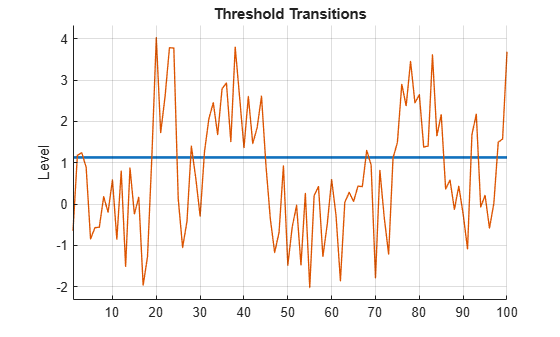`estimate` does not fit the delay parameter $\mathit{d}$ to the data; you must specify its value when you call `estimate`. This example shows how to tune $\mathit{d}$.

Create the following fully specified SETAR model for the DGP.

• State 1: ${\mathit{y}}_{\mathit{t}}={\epsilon }_{1,\mathit{t}}$, where ${\epsilon }_{1,\mathit{t}}\sim Ν\left(0,1\right)$.

• State 2: ${\mathit{y}}_{\mathit{t}}=2+{\epsilon }_{2,\mathit{t}}$, where ${\epsilon }_{2,\mathit{t}}\sim Ν\left(0,2\right)$.

• The system is in state 1 when ${\mathit{y}}_{\mathit{t}-4}<0$, and it is in state 2 otherwise.

```ttDGP = threshold(0); mdl1DGP = arima(Constant=0,Variance=1); mdl2DGP = arima(Constant=2,Variance=2); mdlDGP = [mdl1DGP; mdl2DGP]; MdlDGP = tsVAR(ttDGP,mdlDGP);```

Generate a random response path of length 200 from the DGP. Specify that the threshold variable delay is `4`.

```rng(1) % For reproducibiliy y = simulate(MdlDGP,200,Delay=4); plot(y)```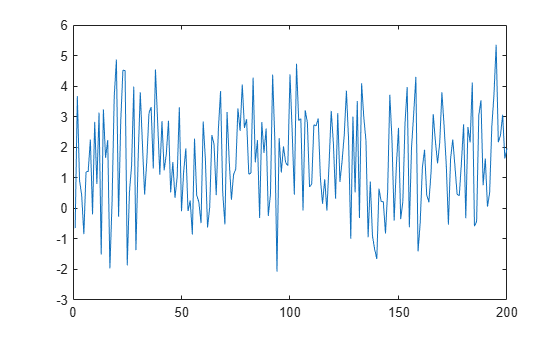Create a partially specified threshold-switching model that has the same structure as the data-generating process, but specify the transition mid-level, submodel coefficients, and state-specific variances as unknown for estimation.

```tt = threshold(NaN); mdl = arima(0,0,0); Mdl = tsVAR(tt,[mdl; mdl]);```

Fully specify a threshold transition that has the same structure as tt, but set the mid-level to `0.5`.

`tt0 = threshold(0.5);`

Tune $\mathit{d}$ by choosing a set of plausible values for it, and by fitting the SETAR model to the simulated data for each value in the set. Choose the model that minimizes the loglikelihood.

```d = 1:8; nd = numel(d); logL = zeros(nd,1); % Preallocate for loglikelihoods EstMdl = cell(nd,1); % Preallocate for estimated models for j = 1:nd [EstMdl{j},logL(j)] = estimate(Mdl,tt0,y,Delay=d(j)); end```

Extract the model that minimizes the loglikelihood.

`[~,optDelay] = max(logL)`
```optDelay = 4 ```
`OptEstMdl = EstMdl{optDelay};`

The optimal delay is `4`, which matches the delay of the DGP.

Display an estimation summary of the optimal model, and display its estimated switching mechanism.

`summarize(OptEstMdl)`
```Description 1-Dimensional tsVAR Model with 2 Submodels Switch Transition Type: discrete Estimated Levels: -0.063 Fit Effective Sample Size: 196 Number of Estimated Parameters: 2 Number of Constrained Parameters: 0 LogLikelihood: -336.184 AIC: 676.367 BIC: 682.923 Submodels Estimate StandardError TStatistic PValue ________ _____________ __________ __________ State 1 Constant(1) -0.33535 0.24271 -1.3817 0.16707 State 2 Constant(1) 2.0073 0.10647 18.853 2.7794e-79 ```

The estimates are close to the parameters of the DGP.

Create the following fully specified SETAR model for the DGP.

• State 1: $\left[\begin{array}{c}{y}_{1,t}\\ {y}_{2,t}\end{array}\right]=\left[\begin{array}{c}-1\\ -4\end{array}\right]+\left[\begin{array}{cc}-0.5& 0.1\\ 0.2& -0.75\end{array}\right]\left[\begin{array}{c}{y}_{1,t-1}\\ {y}_{2,t-1}\end{array}\right]+\left[\begin{array}{c}{\epsilon }_{1,t}\\ {\epsilon }_{2,t}\end{array}\right]$.

• State 2: $\left[\begin{array}{c}{y}_{1,t}\\ {y}_{2,t}\end{array}\right]=\left[\begin{array}{c}1\\ 4\end{array}\right]+\left[\begin{array}{c}{\epsilon }_{1,t}\\ {\epsilon }_{2,t}\end{array}\right]$.

• State 3:$\left[\begin{array}{c}{y}_{1,t}\\ {y}_{2,t}\end{array}\right]=\left[\begin{array}{c}1\\ 4\end{array}\right]+\left[\begin{array}{cc}0.5& 0.1\\ 0.2& 0.75\end{array}\right]\left[\begin{array}{c}{y}_{1,t-1}\\ {y}_{2,t-1}\end{array}\right]+\left[\begin{array}{c}{\epsilon }_{1,t}\\ {\epsilon }_{2,t}\end{array}\right]$.

• $\left[\begin{array}{c}{\epsilon }_{1,t}\\ {\epsilon }_{2,t}\end{array}\right]\sim {N}_{2}\left(\left[\begin{array}{c}0\\ 0\end{array}\right],\left[\begin{array}{cc}2& -1\\ -1& 1\end{array}\right]\right)$.

• The system is in state 1 when ${\mathit{y}}_{2,\mathit{t}-4}<-3$, the system is in state 2 when $-3\le {\mathit{y}}_{2,\mathit{t}-4}<$3, and the system is in state 3 otherwise.

```t = [-3 3]; ttDGP = threshold(t); constant1 = [-1; -4]; constant2 = [1; 4]; constant3 = [1; 4]; AR1 = [-0.5 0.1; 0.2 -0.75]; AR3 = [0.5 0.1; 0.2 0.75]; Sigma = [2 -1; -1 1]; mdl1DGP = varm(Constant=constant1,AR={AR1}); mdl2DGP = varm(Constant=constant2); mdl3DGP = varm(Constant=constant3,AR={AR3}); mdlDGP = [mdl1DGP; mdl2DGP; mdl3DGP]; MdlDGP = tsVAR(ttDGP,mdlDGP,Covariance=Sigma);```

Generate a random response path of length 500 from the DGP. Specify that second response variable with a delay of 4 as the threshold variable.

```rng(10) % For reproducibiliy y = simulate(MdlDGP,500,Index=2,Delay=4);```

Create a partially specified threshold-switching model that has the same structure as the DGP, but specify the transition mid-level, submodel coefficients, and model-wide covariance as unknown for estimation.

```tt = threshold([NaN; NaN]); mdlar = varm(2,1); mdlc = varm(2,0); Mdl = tsVAR(tt,[mdlar; mdlc; mdlar],Covariance=nan(2));```

Fully specify a threshold transition that has the same structure as `tt`, but set the mid-levels to -1 and 1.

```t0 = [-1 1]; tt0 = threshold(t0);```

Fit the threshold-switching model to the simulated series. Specify the threshold variable ${\mathit{y}}_{2,\mathit{t}-4}$. Plot the loglikelihood after each iteration of the threshold search algorithm.

`EstMdl = estimate(Mdl,tt0,y,IterationPlot=true,Index=2,Delay=4);`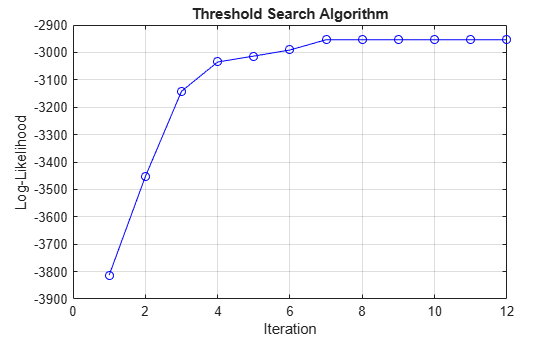The plot displays the evolution of the loglikelihood as the estimation procedure searches for optimal levels. The procedure terminates when one of the stopping criteria is satisfied.

Display an estimation summary of the model.

`summarize(EstMdl)`
```Description 2-Dimensional tsVAR Model with 3 Submodels Switch Transition Type: discrete Estimated Levels: -2.877 2.991 Fit Effective Sample Size: 496 Number of Estimated Parameters: 14 Number of Constrained Parameters: 0 LogLikelihood: -1478.416 AIC: 2984.831 BIC: 3043.723 Submodels Estimate StandardError TStatistic PValue ________ _____________ __________ ___________ State 1 Constant(1) -1.0369 0.13859 -7.4821 7.312e-14 State 1 Constant(2) -4.0179 0.09959 -40.345 0 State 1 AR{1}(1,1) -0.43289 0.063204 -6.8491 7.4332e-12 State 1 AR{1}(2,1) 0.20344 0.045419 4.4791 7.4957e-06 State 1 AR{1}(1,2) 0.076123 0.024477 3.1099 0.0018714 State 1 AR{1}(2,2) -0.75607 0.01759 -42.983 0 State 2 Constant(1) 1.0545 0.14882 7.0858 1.382e-12 State 2 Constant(2) 4.1131 0.10694 38.46 1.9763e-323 State 3 Constant(1) 1.0621 0.095701 11.098 1.2802e-28 State 3 Constant(2) 3.8707 0.068772 56.284 0 State 3 AR{1}(1,1) 0.47396 0.058016 8.1694 3.0997e-16 State 3 AR{1}(2,1) 0.23013 0.041691 5.5199 3.3927e-08 State 3 AR{1}(1,2) 0.10561 0.018233 5.7924 6.9371e-09 State 3 AR{1}(2,2) 0.7568 0.013102 57.761 0 ```

Consider a smooth threshold-switching (STAR) model for the real US GDP growth rate, where each submodel is AR(4) and the threshold variable is the unemployment growth rate.

Create a partially specified threshold transition for the unemployment growth rate. Specify the normal cdf transition function, and an unknown, estimable mid-level and rate. Label the states `"Contraction"` and `"Expansion"`.

```tt = threshold(NaN,Type="normal",Rates=NaN, ... StateNames=["Contraction" "Expansion"]);```

`tt` is a partially specified threshold object, and it is agnostic of the variable and data it represents.

Create a threshold-switching model for the real US GDP growth rate. Label the series `"rRGDP"`.

```mdl = arima(4,0,0); submdls = [mdl; mdl]; Mdl = tsVAR(tt,submdls,SeriesNames="rRGDP");```

Load the quarterly US macroeconomic data set `Data_USEconModel.mat`. Compute the real GDP percent growth and unemployment growth.

```load Data_USEconModel DataTimeTable = rmmissing(DataTimeTable,DataVariables=["GDP" "GDPDEF" "UNRATE"]); RGDP = DataTimeTable.GDP./DataTimeTable.GDPDEF; rRGDP = price2ret(RGDP)*100; % Response data gUNRATE = diff(DataTimeTable.UNRATE); % Exogenous thresold data dates = DataTimeTable.Time(2:end);```

Suppose the estimation period includes growth rates from the first quarter of 1950. Identify the estimation period in the date base.

```startEst = datetime(1950,1,1); idxEst = dates >= startEst;```

To initialize the estimation procedure, fully specify a threshold transition that has the same structure as `tt`, but set the mid-level to `-0.5` with a rate of `100`.

`tt0 = threshold(-0.5,Type=tt.Type,Rates=100,StateNames=tt.StateNames);`

Fit the STAR model to the estimation period of the US GDP growth rate series. Specify the following parameters:

• Set `Y0` to the responses before the estimation period to initialize the AR submodel components.

• Set `Type` to "exogenous" to characterize the threshold variable.

• Set Z to the threshold variable data `gUNRATE` in the estimation period.

• Set `MaxRate` to `150` to expand the search for the optimal transition function rate.

• Plot the evolution of the loglikelihood.

```EstMdl = estimate(Mdl,tt0,rRGDP(idxEst),Y0=rRGDP(~idxEst), ... Z=gUNRATE(idxEst),Type="exogenous",MaxRate=150,IterationPlot=true);```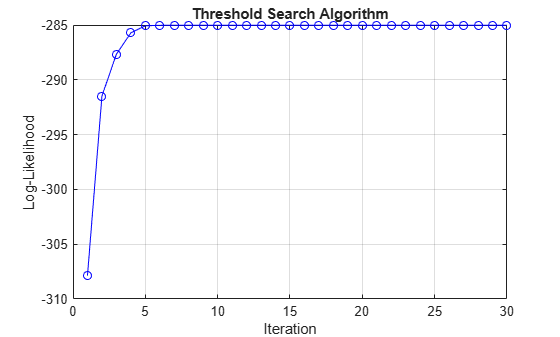Display an estimation summary and plot the estimated switching mechanism with the threshold data.

`summarize(EstMdl)`
```Description 1-Dimensional tsVAR Model with 2 Submodels Switch Transition Type: normal Estimated Levels: 0.208 Estimated Rates: 75.566 Fit Effective Sample Size: 237 Number of Estimated Parameters: 10 Number of Constrained Parameters: 0 LogLikelihood: -285.022 AIC: 590.043 BIC: 624.724 Submodels Estimate StandardError TStatistic PValue _________ _____________ __________ __________ State 1 Constant(1) 1.0473 0.11549 9.068 1.2125e-19 State 1 AR{1}(1,1) 0.15792 0.068783 2.2959 0.021679 State 1 AR{2}(1,1) 0.059888 0.066409 0.9018 0.36716 State 1 AR{3}(1,1) -0.10455 0.070384 -1.4854 0.13744 State 1 AR{4}(1,1) -0.098037 0.063249 -1.55 0.12114 State 2 Constant(1) -0.12491 0.15383 -0.81197 0.41681 State 2 AR{1}(1,1) 0.15366 0.13993 1.0981 0.27215 State 2 AR{2}(1,1) -0.027925 0.16754 -0.16667 0.86763 State 2 AR{3}(1,1) -0.24366 0.12778 -1.9068 0.056543 State 2 AR{4}(1,1) -0.075389 0.14024 -0.53759 0.59086 ```
```figure ttplot(EstMdl.Switch,Data=gUNRATE(idxEst)) dEst = dates(idxEst); xticklabels(string(dEst(xticks)))```The large rate suggests little mixing occurs between the models of each regime. When the quarterly unempoyment growth is less than 0.206%, the dominant model for the US GDP growth rate is in `EstMdl.Submodels(1)`. Otherwise, the dominant submodel is in `EstMdl.Submodels(2)`.

Many of the coefficients are insignificant, which can suggest a search for a simpler model.

This example shows how to specify equality constraints on estimable submodel coefficients and threshold parameters.

Consider the model for the real US GDP growth rate in Specify Presample Data for STAR Model Estimation. Assume the submodels are AR(4), but they include only the model constant (time trend in differenced series) and fourth lag. Also, suppose the transition function rate 3 for the unemployment growth series.

Create the partially specified threshold transition for the unemployment growth rate. Specify the known transition rate.

```rConstraint = 3; tt = threshold(NaN,Type="normal",Rates=rConstraint, ... StateNames=["Contraction" "Expansion"]);```

Create a threshold-switching model for the real US GDP growth rate. Explicitly specify the AR lags to include in the submodels by using the `ARLags` option.

```mdl = arima(ARLags=4); submdls = [mdl; mdl]; Mdl = tsVAR(tt,submdls,SeriesNames="rRGDP");```

Load the quarterly US macroeconomic data set `Data_USEconModel.mat`. Compute the real GDP percent growth and unemployment growth.

```load Data_USEconModel DataTimeTable = rmmissing(DataTimeTable,DataVariables=["GDP" "GDPDEF" "UNRATE"]); RGDP = DataTimeTable.GDP./DataTimeTable.GDPDEF; rRGDP = price2ret(RGDP)*100; % Response data gUNRATE = diff(DataTimeTable.UNRATE); % Exogenous thresold data dates = DataTimeTable.Time(2:end);```

Suppose the estimation period includes growth rates from the first quarter of 1950. Identify the estimation period in the date base.

```startEst = datetime(1950,1,1); idxEst = dates >= startEst;```

Fully specify a threshold transition that has the same structure as `tt`, including the equality constraint on the transition function rate. Set the initial mid-level to 0.

`tt0 = threshold(0,Type=tt.Type,Rates=rConstraint,StateNames=tt.StateNames);`

Fit the STAR model to the estimation period of the US GDP growth rate series. Specify the following parameters:

• Set `Y0` to the responses before the estimation period to initialize the AR submodel components.

• Set `Type` to `"exogenous"` to characterize the threshold variable.

• Set `Z` to the threshold variable data `gUNRATE` in the estimation period.

• Set `MaxRate` to 150 to expand the search for the optimal transition function rate.

• Plot the evolution of the loglikelihood.

```EstMdl = estimate(Mdl,tt0,rRGDP(idxEst),Y0=rRGDP(~idxEst), ... Z=gUNRATE(idxEst),Type="exogenous",IterationPlot=true);```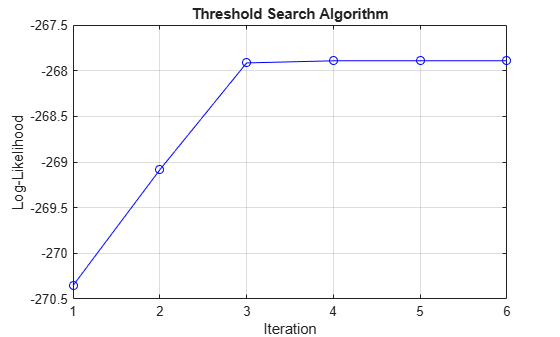Display an estimation summary and plot the estimated switching mechanism with the threshold data.

`summarize(EstMdl)`
```Description 1-Dimensional tsVAR Model with 2 Submodels Switch Transition Type: normal Estimated Levels: 0.228 Estimated Rates: 3.000 Fit Effective Sample Size: 237 Number of Estimated Parameters: 4 Number of Constrained Parameters: 6 LogLikelihood: -267.892 AIC: 543.784 BIC: 557.656 Submodels Estimate StandardError TStatistic PValue _________ _____________ __________ __________ State 1 Constant(1) 1.5928 0.087805 18.14 1.543e-73 State 1 AR{1}(1,1) 0 0 NaN NaN State 1 AR{2}(1,1) 0 0 NaN NaN State 1 AR{3}(1,1) 0 0 NaN NaN State 1 AR{4}(1,1) -0.11789 0.067607 -1.7438 0.081194 State 2 Constant(1) -0.73667 0.15808 -4.6601 3.1609e-06 State 2 AR{1}(1,1) 0 0 NaN NaN State 2 AR{2}(1,1) 0 0 NaN NaN State 2 AR{3}(1,1) 0 0 NaN NaN State 2 AR{4}(1,1) -0.040337 0.1299 -0.31053 0.75616 ```
```figure ttplot(EstMdl.Switch,Data=gUNRATE(idxEst)) dEst = dates(idxEst); xticklabels(string(dEst(xticks)))```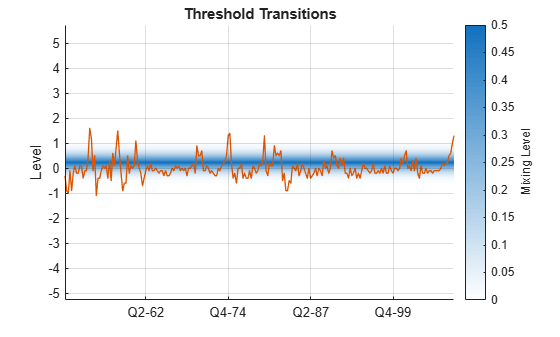Consider the following data-generating process (DGP) for a 2-D time-varying TAR (TVTAR) model containing an exogenous regression component.

• State 1: ${y}_{t}=\left[\begin{array}{c}1\\ -1\end{array}\right]+\left[\begin{array}{cc}0.6& 0.1\\ 0.4& 0.2\end{array}\right]{y}_{t-1}+{x}_{t}\left[\begin{array}{c}0.2\\ -0.4\end{array}\right]+{\epsilon }_{1,t}$, where ${\epsilon }_{1,\mathit{t}}\sim {Ν}_{2}\left(0,0.5{\mathit{I}}_{2}\right)$ and ${\mathit{I}}_{2}$ is the 2-by-2 identity matrix.

• State 2: ${y}_{t}=\left[\begin{array}{c}2\\ -2\end{array}\right]+\left[\begin{array}{cc}0.6& 0.1\\ 0.4& 0.2\end{array}\right]{y}_{t-1}+{x}_{t}\left[\begin{array}{c}0.6\\ -1.0\end{array}\right]+{\epsilon }_{2,t}$, where ${\epsilon }_{2,\mathit{t}}\sim {Ν}_{2}\left(0,{\mathit{I}}_{2}\right)$.

• State 3: ${y}_{t}=\left[\begin{array}{c}3\\ -3\end{array}\right]+\left[\begin{array}{cc}0.6& 0.1\\ 0.4& 0.2\end{array}\right]{y}_{t-1}+{x}_{t}\left[\begin{array}{c}0.9\\ -1.3\end{array}\right]+{\epsilon }_{3,t}$, where ${\epsilon }_{3,\mathit{t}}\sim {Ν}_{2}\left(0,1.5{\mathit{I}}_{2}\right)$.

• The exogenous threshold variable ${\mathit{z}}_{\mathit{t}}$ represents a linear time trend over the sample period. In this example, ${\mathit{z}}_{\mathit{t}}$ is the sampling period normalized by the sample size.

• The system is in state 1 when ${\mathit{z}}_{\mathit{t}}<0.3$, the system is in state 2 when $0.3\le {\mathit{z}}_{\mathit{t}}<0.6$, and the system is in state 3 otherwise.

Create a TVTAR model that represents the DGP.

```tDGP = [0.3 0.6]; ttDGP = threshold(tDGP); % Constant vectors C1 = [1;-1]; C2 = [2;-2]; C3 = [3;-3]; % Common Autoregression coefficient AR = {[0.6 0.1; 0.4 0.2]}; % Regression coefficient vectors Beta1 = [0.2;-0.4]; Beta2 = [0.6;-1.0]; Beta3 = [0.9;-1.3]; % Innovations covariance matrices Sigma1 = 0.5*eye(2); Sigma2 = eye(2); Sigma3 = 1.5*eye(2); % VAR Submodels mdl1 = varm(Constant=C1,AR=AR,Beta=Beta1,Covariance=Sigma1); mdl2 = varm(Constant=C2,AR=AR,Beta=Beta2,Covariance=Sigma2); mdl3 = varm(Constant=C3,AR=AR,Beta=Beta3,Covariance=Sigma3); mdlDGP = [mdl1; mdl2; mdl3]; DGP = tsVAR(ttDGP,mdlDGP);```

For the exogenous predictors ${\mathit{x}}_{\mathit{t}}$, simulate a 2-D 1000-period path from the Gaussian distribution with mean 0 and standard deviation 10.

```rng(1); % For reproducibility numPeriods = 1000; X = 10*randn(numPeriods,2);```

For the threshold variable, create a 1000-element vector of equally spaced elements from 0 to 1.

`z = linspace(0,1,numPeriods)';`

Simulate a 1000-period path from the DGP.

`Y = simulate(DGP,numPeriods,X=X,Type="exogenous",Z=z);`

Create a TVTAR model template for estimation. Specify all estimable parameters as unknown by using `NaN`s.

```t = [NaN NaN]; tt = threshold(t); mdl = varm(2,1); % Unknown contsant and AR coefficient by default mdl.Beta = NaN(2,1); % Configure exogenous regression component for estimation Mdl = tsVAR(tt,[mdl; mdl; mdl]);```

Specify initial values of 0.25 and 0.7 for the regime thresholds.

```t0 = [.25 .7]; tt0 = threshold(t0);```

The largest lag among all models is 1. Therefore, the estimation procedure requires one presample observation to initialize the model.

Identify indices for the required presample, and identify indices for the estimation sample.

```p = mdl.P; idxPre = 1:p; idxEst = (p + 1):numPeriods;```

Fit the TVTAR model to the data. Specify presample responses `Y0`, characterize the threshold variable `Type` and provide its data `Z`, and specify the exogenous data `X`.

```EstMdl = estimate(Mdl,tt0,Y(idxEst,:),Y0=Y(idxPre,:), ... Type="exogenous",Z=z(idxEst),X=X(idxEst,:));```

Display an estimation summary separately for each state, and display the estimated threshold transitions.

`summarize(EstMdl,1)`
```Description 2-Dimensional VAR Submodel, State 1 Submodel Estimate StandardError TStatistic PValue ________ _____________ __________ ___________ State 1 Constant(1) 0.6284 0.1463 4.2952 1.7456e-05 State 1 Constant(2) -0.86147 0.1664 -5.1771 2.2538e-07 State 1 AR{1}(1,1) 0.64569 0.046378 13.922 4.6483e-44 State 1 AR{1}(2,1) 0.41777 0.052749 7.92 2.3752e-15 State 1 AR{1}(1,2) 0.11447 0.029747 3.8481 0.00011901 State 1 AR{1}(2,2) 0.18968 0.033833 5.6064 2.0657e-08 State 1 Beta(1,1) 0.12661 0.01005 12.598 2.1562e-36 State 1 Beta(2,1) -0.28306 0.01143 -24.765 2.1435e-135 ```
`summarize(EstMdl,2)`
```Description 2-Dimensional VAR Submodel, State 2 Submodel Estimate StandardError TStatistic PValue ________ _____________ __________ __________ State 2 Constant(1) 2.0016 0.20741 9.6503 4.9029e-22 State 2 Constant(2) -1.919 0.2359 -8.1345 4.1377e-16 State 2 AR{1}(1,1) 0.59692 0.033401 17.871 1.9848e-71 State 2 AR{1}(2,1) 0.37274 0.03799 9.8117 1.0027e-22 State 2 AR{1}(1,2) 0.1173 0.023148 5.0674 4.0337e-07 State 2 AR{1}(2,2) 0.18002 0.026327 6.8376 8.0532e-12 State 2 Beta(1,1) 0.6689 0.014264 46.896 0 State 2 Beta(2,1) -1.122 0.016223 -69.16 0 ```
`summarize(EstMdl,3)`
```Description 2-Dimensional VAR Submodel, State 3 Submodel Estimate StandardError TStatistic PValue ________ _____________ __________ ___________ State 3 Constant(1) 3.1135 0.10796 28.841 6.5868e-183 State 3 Constant(2) -3.135 0.12279 -25.533 8.5619e-144 State 3 AR{1}(1,1) 0.61547 0.011379 54.089 0 State 3 AR{1}(2,1) 0.40065 0.012942 30.958 1.9801e-210 State 3 AR{1}(1,2) 0.10685 0.0089014 12.004 3.4042e-33 State 3 AR{1}(2,2) 0.19124 0.010124 18.889 1.3979e-79 State 3 Beta(1,1) 0.92011 0.0080742 113.96 0 State 3 Beta(2,1) -1.3378 0.0091834 -145.68 0 ```
`EstMdl.Switch`
```ans = threshold with properties: Type: 'discrete' Levels: [0.3096 0.5887] Rates: [] StateNames: ["1" "2" "3"] NumStates: 3 ```

## Input Arguments

collapse all

Partially specified threshold-switching dynamic regression model used to indicate constrained and estimable model parameters, specified as a `tsVAR` model object returned by `tsVAR`. Properties of `Mdl` describe the model structure and specify the parameters.

`estimate` treats specified parameters as equality constraints during estimation.

`estimate` fits unspecified (`NaN`-valued) parameters to the data `Y`.

Because `estimate` computes innovations covariances after estimation, you cannot constrain them. If `Mdl.Covariance` is nonempty (see `tsVAR`), `estimate` provides an estimate in `EstMdl.Covariance`. Otherwise, for each `i` in `1:Mdl.NumStates`, `estimate` provides the innovations covariance estimate of state `i` in `EstMdl.Submodels(i).Covariance`.

Fully specified threshold transitions used to initialize the estimation procedure, specified as a `threshold` object returned by `threshold`. Properties of a fully specified model object do not contain `NaN` values.

`tt0` is a copy of `Mdl.Switch` with `NaN` values replaced by initial values for levels (`Mdl.Switch.Levels`) and rates (`Mdl.Switch.Rates`). Levels (`tt0.Levels`) and rates (`tt0.Rates`) must be within the limits set by the name-value arguments `'MaxLevel'` and `'MaxRate'`.

Tip

For broad coverage of the parameter space, run the algorithm from multiple instances of `tt0`. For more details, see Algorithms.

Observed response data to which `estimate` fits the model, specified as a `numObs`-by-`numSeries` numeric matrix.

`numObs` is the sample size. `numSeries` is the number of response variables (`Mdl.NumSeries`).

Rows correspond to observations, and the last row contains the latest observation. Columns correspond to individual response variables.

`Y` represents the continuation of the presample response series in `Y0`.

Data Types: `double`

Axes on which to plot the loglikelihood for each iteration when the `'IterationPlot'` name-value argument is `true`, specified as an `Axes` object.

By default, `estimate` plots to the current axes (`gca`).

If `'IterationPlot'` is `false`, `estimate` ignores `ax`.

### Name-Value Arguments

Specify optional pairs of arguments as `Name1=Value1,...,NameN=ValueN`, where `Name` is the argument name and `Value` is the corresponding value. Name-value arguments must appear after other arguments, but the order of the pairs does not matter.

Before R2021a, use commas to separate each name and value, and enclose `Name` in quotes.

Example: `Type="exogenous",Z=z,IterationPlot=true` specifies data `z` for the exogenous threshold variable and plots the loglikelihood for each iteration.

Type of threshold variable data, specified as a value in this table.

ValueDescription
`"endogenous"`

The model is self-exciting with threshold variable data ${z}_{t}={y}_{j,\left(t-d\right)},$ generated by response j, where

`"exogenous"`The threshold variable is exogenous to the system. The name-value argument `'Z'` specifies the threshold variable data and is required.

Example: `Type="exogenous",Z=z` specifies the data `z` for the exogenous threshold variable.

Example: `Type="endogenous",Index=2,Delay=4` specifies the endogenous threshold variable as y2,t−4, whose data is `Y(:,2)`.

Data Types: `char` | `string` | `cell`

Presample response data, specified as a `numPreSampleObs`-by-`numSeries` numeric matrix.

The number of presample observations `numPreSampleObs` must be sufficient to initialize the AR terms of all submodels. For models of type `"endogenous"`, the number of presample observations must also be sufficient to initialize the delayed response. If `numPreSampleObs` exceeds the number necessary to initialize the model, `estimate` uses only the latest observations. The last row contains the latest observations.

By default, `Y0` is the initial segment of `Y`, which reduces the effective sample size.

Data Types: `double`

Threshold variable data for models of type `"exogenous"`, specified as a numeric vector of length at least `numObs`. If the length exceeds `numobs`, `estimate` uses only the latest observations. The last row contains the latest observation.

Data Types: `double`

Threshold variable delay d in yj,td, for models of type `"endogenous"`, specified as a positive integer.

Example: `Delay=4` specifies that the threshold variable is y2,td, where j is the value of `Index`

.

Data Types: `double`

Threshold variable index j in yj,td for models of type `"endogenous"`, specified as a scalar in `1:Mdl.NumSeries`.

`estimate` ignores `Index` for univariate AR models.

Example: `Index=2` specifies that the threshold variable is y2,td, where d is the value of `Delay`.

Data Types: `double`

Predictor data used to evaluate regression components in all submodels of `Mdl`, specified as a numeric matrix or a cell vector of numeric matrices.

To use a subset of the same predictors in each state, specify `X` as a matrix with `numPreds` columns and at least `numObs` rows. Columns correspond to distinct predictor variables. Submodels use initial columns of the associated matrix, in order, up to the number of submodel predictors. The number of columns in the `Beta` property of `Mdl.SubModels(j)` determines the number of exogenous variables in the regression component of submodel `j`. If the number of rows exceeds `numObs`, then `estimate` uses the latest observations.

To use different predictors in each state, specify a cell vector of such matrices with length `numStates`.

By default, `estimate` ignores regression components in `Mdl`.

Data Types: `double`

Maximum deviation of estimated threshold levels above or below the threshold variable data, z or yj,td, specified as a positive numeric scalar representing the percent of the range.

The default value `0` means that initial and estimated levels must be within the range of the data. A value greater than `0` expands the range of the search.

Example: `MaxLevel=10` expands the search for levels 10% above and below the range of the threshold variable data.

Data Types: `double`

Maximum estimated threshold transition rates, specified as a positive numeric scalar or vector with length equal to the number of rates in `Mdl.Switch.Rates`.

For a scalar, all estimated rates are bounded by `MaxRate`. For a vector, the estimate of `Mdl.Switch.Rate(j)` is bounded by `MaxRate(j)`.

`estimate` estimates discrete transition levels as logistic transitions at `MaxRate`.

The default value is `10` for each rate.

Example: `MaxLevel=3` bounds all estimated rates in `Mdl.Switch.Rates` by `3`.

Example: `MaxLevel=[5 10]` bounds `Mdl.Switch.Rates(1)` by `5` and bounds `Mdl.Switch.Rates(2)` by `10`.

Data Types: `double`

Limit on the number of iterations of the threshold search algorithm, specified as a positive integer.

Example: `MaxIterations=1000`

Data Types: `double`

Convergence tolerance, specified as a positive numeric scalar. The search algorithm terminates after an iteration in which the optimality tolerance on loglikelihood objective `logL` changes by less than the value of `Tolerance`.

Example: `Tolerance=1e-4`

Data Types: `double`

Flag indicating whether to show a plot of the loglikelihood versus iteration step at algorithm termination, specified as a value in this table.

ValueDescription
`true`When the algorithm terminates, `estimate` shows a plot of the loglikelihood at each iteration step.
`false``estimate` does not show a plot.

Example: `IterationPlot=true`

Data Types: `logical`

## Output Arguments

collapse all

Estimated threshold-switching dynamic regression model, returned as a `tsVAR` object. `EstMdl` is a copy of `Mdl` that has `NaN` values replaced with parameter estimates. `EstMdl` is fully specified.

Estimated loglikelihood of the data in `Y` from the conditional least-squares problem, returned as a numeric scalar and evaluated at the optimal parameter values in the threshold transitions `EstMdl.Switch`. For more details, see Algorithms.

Handle to the iteration plot, returned as a graphics object when `IterationPlot` is `true`.

`h` contains a unique plot identifier, which you can use to query or modify properties of the plot.

## Tips

• Several factors can lead to poor estimates of model parameters. The factors include:

• Threshold data does not cross levels or sufficiently sample submodels.

• `Mdl` contains more estimable parameters than the sample size supports.

• High-rate transitions are indistinguishable.

• Self-exciting autoregressive models have multiple sources of endogenous dynamics.

To improve estimates, perform these actions:

• Control the parameter search by experimenting with the `'MaxLevel'` and `'MaxRate'` name-value arguments.

• Consider a parsimonious model with initial levels within the range of threshold variable data.

• Constrain specific parameters to potentially improve performance.

• To estimate the delay d in a self-exciting model, compare the loglikelihood `logL` with different specifications for the `'Delay'` name-value argument. In practice, usually d is limited to a range of reasonable values.

• You can estimate a simple time-varying STAR (TVSTAR) model by specifying exogenous threshold data z = t, which is a linear time trend over the sample period .

## Algorithms

• `estimate` searches over levels and rates for `EstMdl.Switch` while solving a conditional least-squares problem for submodel parameters, maximizing the data likelihood, as described in . `logL` is conditional on the optimal parameter values in `EstMdl.Switch`.

• Models with smooth transitions (STAR models) represent the response as a weighted mixture of conditional means from all submodels (, Eqn. 3.6). `estimate` determines the weights by the value of the threshold variable, z or yj,td, relative to threshold levels. `estimate` treats models with discrete transitions (TAR models) as logistic STAR models with transition rates set to `'MaxRate'` in order to disambiguate search levels that fall between threshold variable data.

• `estimate` handles two types of equality constraints.

`estimate` computes innovations variances and covariances after estimation. Therefore, you cannot constrain them.

 Chan, Kung-Sik, and Howell Tong. “On Estimating Thresholds in Autoregressive Models.” Journal of Time Series Analysis 7 (May 1986): 179–90. https://doi.org/10.1111/j.1467-9892.1986.tb00501.x.

 Hamilton, James D. Time Series Analysis. Princeton, NJ: Princeton University Press, 1994.

 Teräsvirta, Tima. "Modelling Economic Relationships with Smooth Transition Regressions." In A. Ullahand and D.E.A. Giles (eds.), Handbook of Applied Economic Statistics, 507–552. New York: Marcel Dekker, 1998.

 van Dijk, Dick. Smooth Transition Models: Extensions and Outlier Robust Inference. Rotterdam, Netherlands: Tinbergen Institute Research Series, 1999.